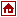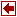## Linear Programming Packages

 LPAKO Author Prof. Park Soondal and the members of System Analysis Laboratory (Operations Research lab.) in Dept. of Industrial Engineering Seoul National University, Shillim-dong, Kwanak-gu Seoul, Korea 151-742 Tel: +82-2-880-7183 E-mail: orlab@orlab.snu.ac.kr Language C++ Algorithm simplex Input Format MPS Modeling Languages link Commercial Status free Platform PC, UNIX Remarks This program can solve the following linear programming problems; Max C^T X s.t. d <= AX <= b L <= X <= U. It solves problems by the simplex method using the following techniques: Pricing strategy: Dynamic Steepest-edge simplex method with full pricing . Maintenace of basis: LU factorization with Markowitz ordering. Basis matrix update: Modified Forrest-Tomlin LU update method. Anti-degeneracy mechanism: A variation of EXPAND Procedure. Preprocessing & Scaling. This program uses the revised simplex method to solve the linear programming problems. Especially, uses the LU (Markowitz' strategy) decomposition form to store basic matrices and the two-phase method to get an initial BFS. The program uses multiple pricing and partial pricing. The global variable MPRICE determines the number of candidates as entering variables and the minor iterations are excecuted until these candidates are exhausted. After this the major iteration is excecuted. This program uses modified Forrest-Tomlin's basis updating method. This method performs row-column permutations to reduce the number of fill-ins and maintain the sparsity and the numerical stability of basic matrix. References